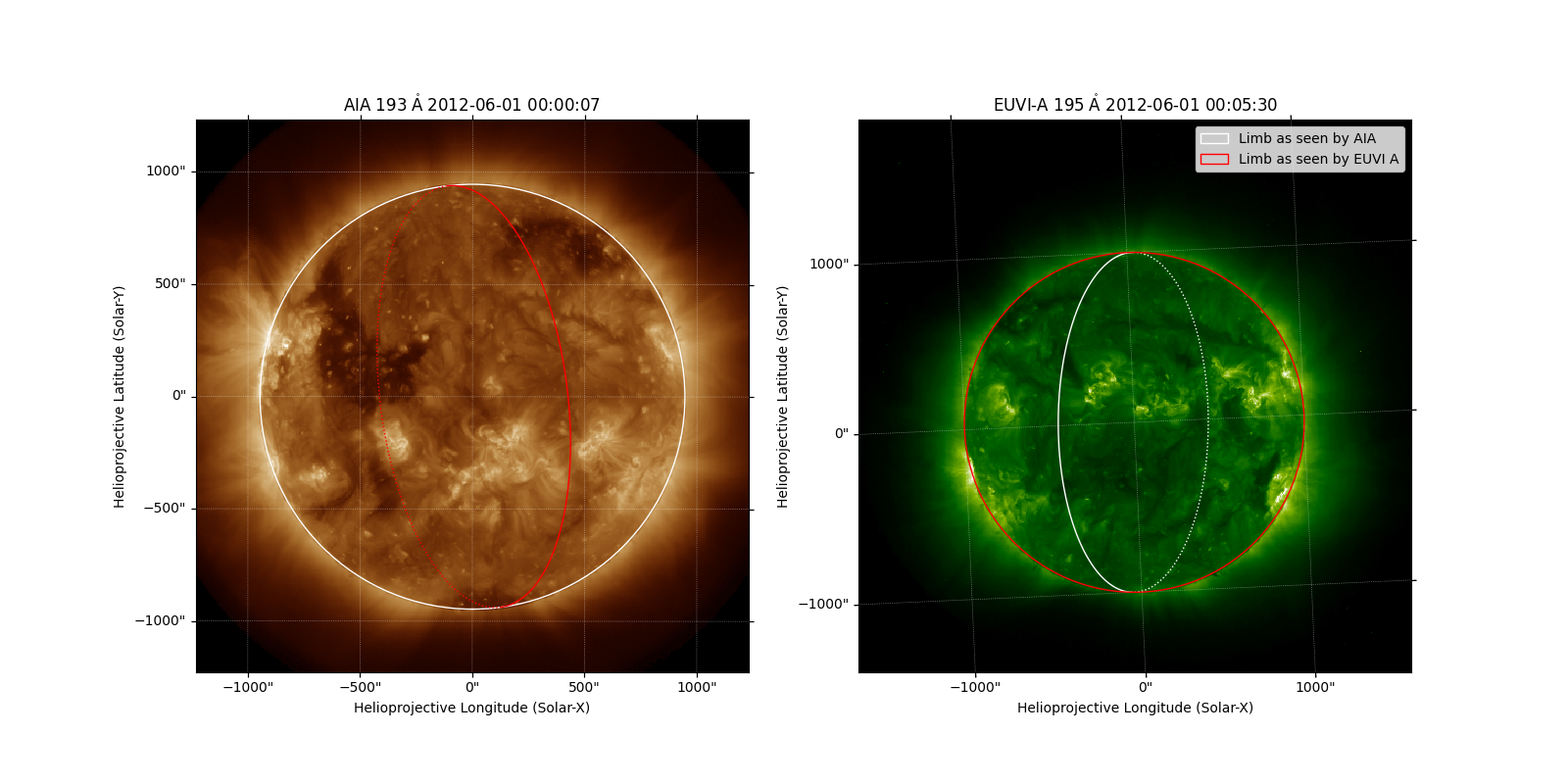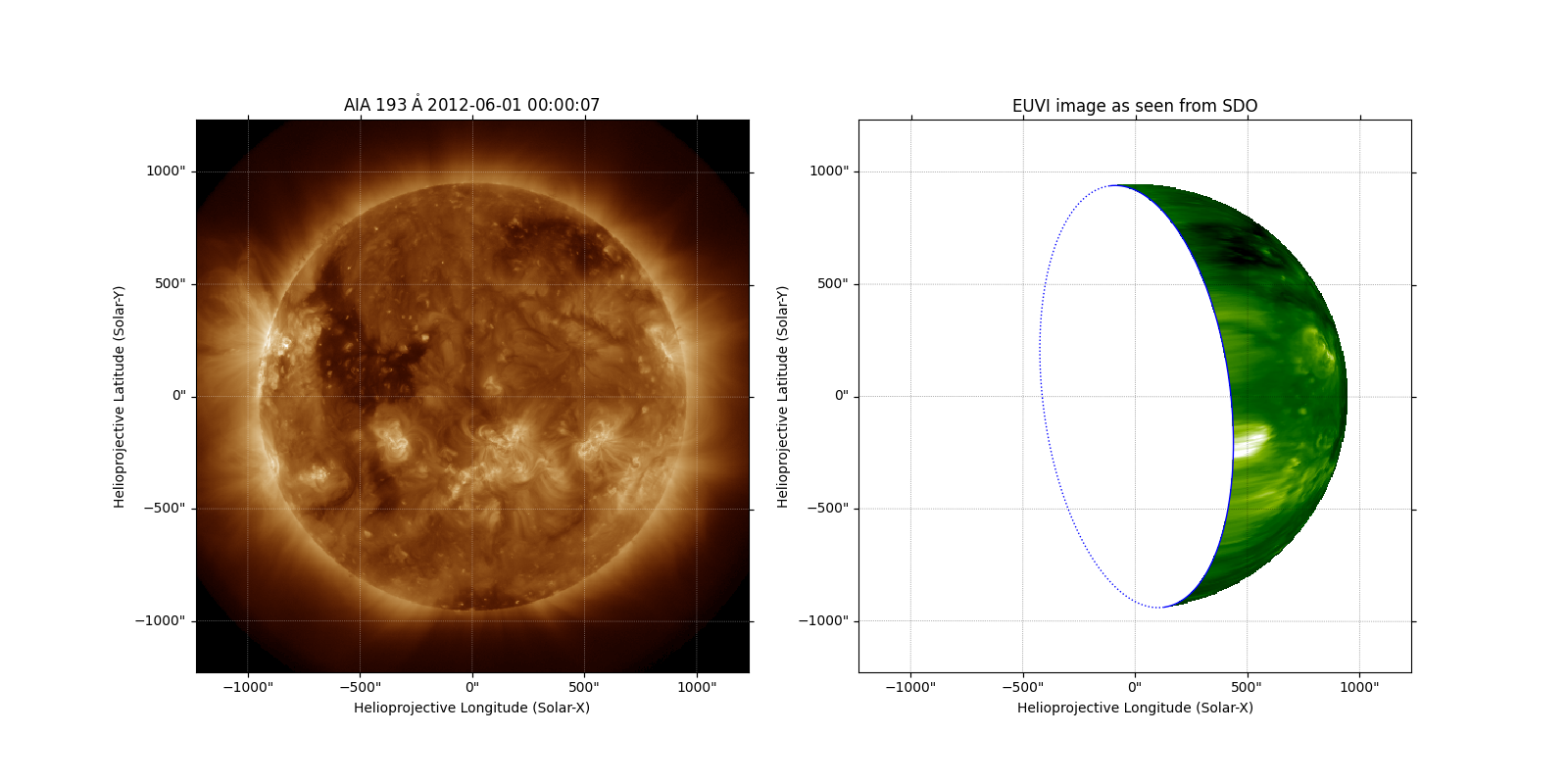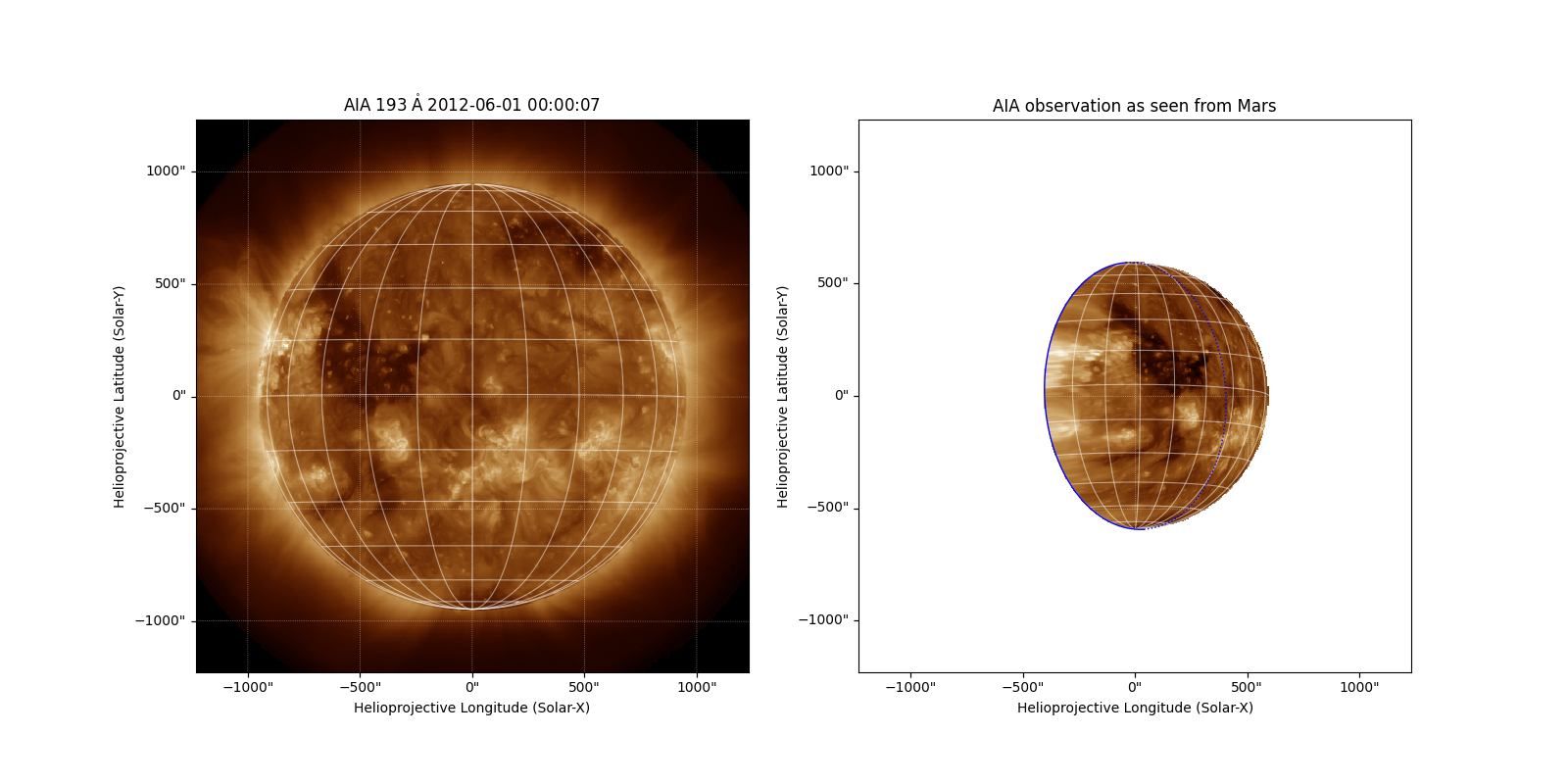# Reprojecting Images to Different Observers¶

This example demonstrates how you can reproject images to the view from different observers. We use data from these two instruments:

• AIA on SDO, which is in orbit around Earth

• EUVI on STEREO A, which is in orbit around the Sun away from the Earth

You will need reproject v0.6 or higher installed.

import matplotlib.pyplot as plt

import astropy.units as u
from astropy.coordinates import SkyCoord

import sunpy.map
from sunpy.coordinates import get_body_heliographic_stonyhurst
from sunpy.data.sample import AIA_193_JUN2012, STEREO_A_195_JUN2012


In this example we are going to make a lot of side by side figures, so let’s change the default figure size.

plt.rcParams['figure.figsize'] = (16, 8)


Create a map for each image, after making sure to sort by the appropriate name attribute (i.e., “AIA” and “EUVI”) so that the order is reliable.

map_list = sunpy.map.Map([AIA_193_JUN2012, STEREO_A_195_JUN2012])
map_list.sort(key=lambda m: m.detector)
map_aia, map_euvi = map_list

# We downsample these maps to reduce memory consumption, but you can
# comment this out.
out_shape = (512, 512)
map_aia = map_aia.resample(out_shape * u.pix)
map_euvi = map_euvi.resample(out_shape * u.pix)


Plot the two maps, with the solar limb as seen by each observatory overlaid on both plots.

fig = plt.figure()

map_aia.plot(axes=ax1)
map_aia.draw_limb(axes=ax1, color='white')
map_euvi.draw_limb(axes=ax1, color='red')

map_euvi.plot(axes=ax2)
limb_aia = map_aia.draw_limb(axes=ax2, color='white')
limb_euvi = map_euvi.draw_limb(axes=ax2, color='red')

plt.legend([limb_aia, limb_euvi],
['Limb as seen by AIA', 'Limb as seen by EUVI A'])INFO: Missing metadata for solar radius: assuming the standard radius of the photosphere. [sunpy.map.mapbase]

<matplotlib.legend.Legend object at 0x7f7b159d3e20>


We now need to construct an output WCS. We build a custom header using sunpy.map.header_helper.make_fitswcs_header() but we use a lot of the map_aia properties to do it.

out_header = sunpy.map.make_fitswcs_header(
out_shape,
map_aia.reference_coordinate.replicate(rsun=map_euvi.reference_coordinate.rsun),
scale=u.Quantity(map_aia.scale),
instrument="EUVI",
observatory="AIA Observer",
wavelength=map_euvi.wavelength
)


We can now reproject the EUVI map to this output WCS header. The reproject_to() defaults to using the fast reproject.reproject_interp() algorithm, but a different algorithm can be specified (e.g., reproject.reproject_adaptive()).

outmap = map_euvi.reproject_to(out_header)


We can now plot the STEREO/EUVI image as seen from the position of SDO, next to the AIA image.

fig = plt.figure()
map_aia.plot(axes=ax1)
outmap.plot(axes=ax2, title='EUVI image as seen from SDO')
map_euvi.draw_limb(color='blue')

# Set the HPC grid color to black as the background is white
ax2.coords.grid_lines_kwargs['edgecolor'] = 'k'
ax2.coords.grid_lines_kwargs['edgecolor'] = 'k'INFO: Missing metadata for solar radius: assuming the standard radius of the photosphere. [sunpy.map.mapbase]


## AIA as Seen from Mars¶

The new observer coordinate doesn’t have to be associated with an existing Map. sunpy provides a function which can get the location coordinate for any known body. In this example, we use Mars.

mars = get_body_heliographic_stonyhurst('mars', map_aia.date)


To generate a target WCS, we first need an appropriate reference coordinate, which is similar to the one for AIA, except now with the observer at Mars.

mars_ref_coord = SkyCoord(0*u.arcsec, 0*u.arcsec,
obstime=map_aia.reference_coordinate.obstime,
observer=mars,
rsun=map_aia.reference_coordinate.rsun,
frame="helioprojective")


We then create the WCS header.

mars_header = sunpy.map.make_fitswcs_header(
out_shape,
mars_ref_coord,
scale=u.Quantity(map_aia.scale),
rotation_matrix=map_aia.rotation_matrix,
instrument="AIA",
wavelength=map_aia.wavelength
)


We generate the output map and plot it next to the original image.

outmap = map_aia.reproject_to(mars_header)

fig = plt.figure()

map_aia.plot(axes=ax1)
map_aia.draw_grid(color='w')